# 使用VisualVM分析性能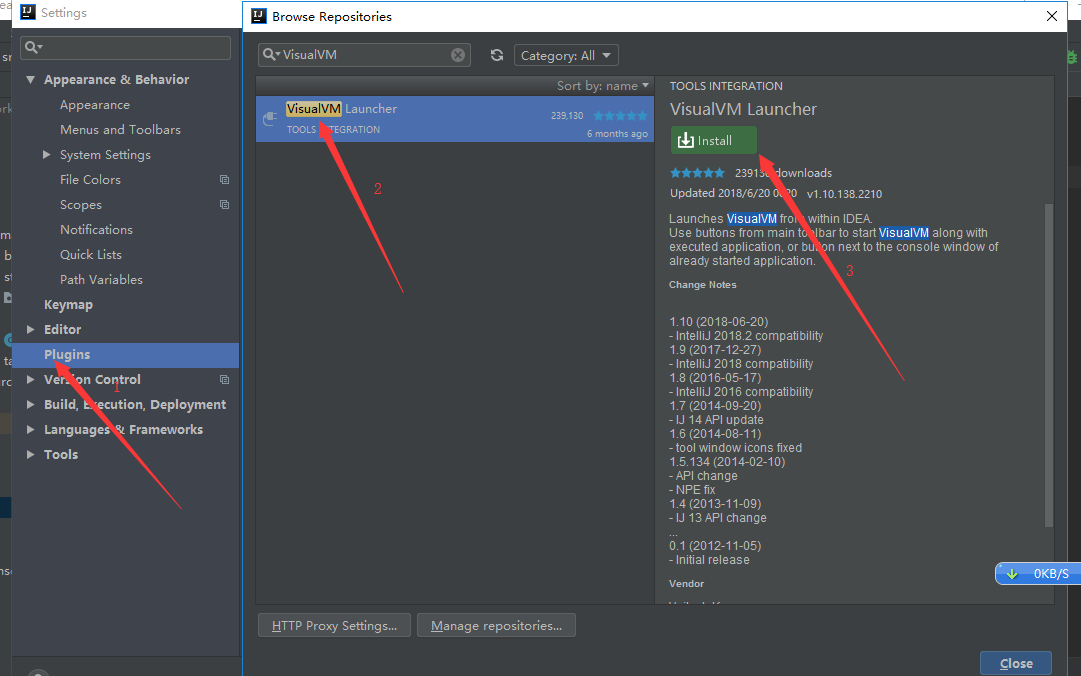## 一、查看程序的JVM内存

public class JavaHeapTest {
public final static int OUTOFMEMORY = 200000000;

private String oom;

private int length;

StringBuffer tempOOM = new StringBuffer();

public JavaHeapTest(int leng) {
this.length = leng;

int i = 0;
while (i < leng) {
i++;
try {
tempOOM.append("a");
} catch (OutOfMemoryError e) {
e.printStackTrace();
break;
}
}
this.oom = tempOOM.toString();

}

public String getOom() {
return oom;
}

public int getLength() {
return length;
}

public static void main(String[] args) {
JavaHeapTest javaHeapTest = new JavaHeapTest(OUTOFMEMORY);
System.out.println(javaHeapTest.getOom().length());
}

}


然后使用VIsualVM运行程序。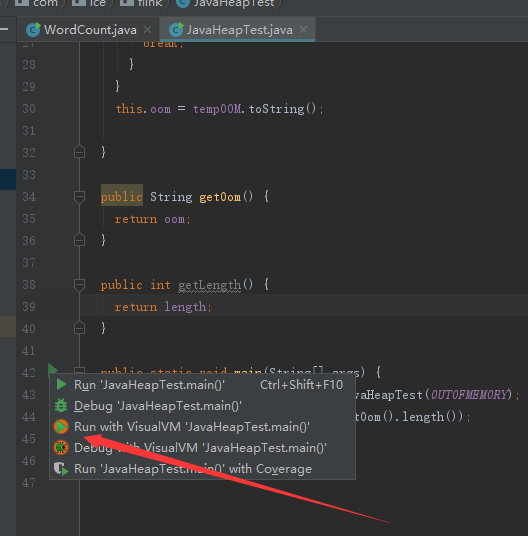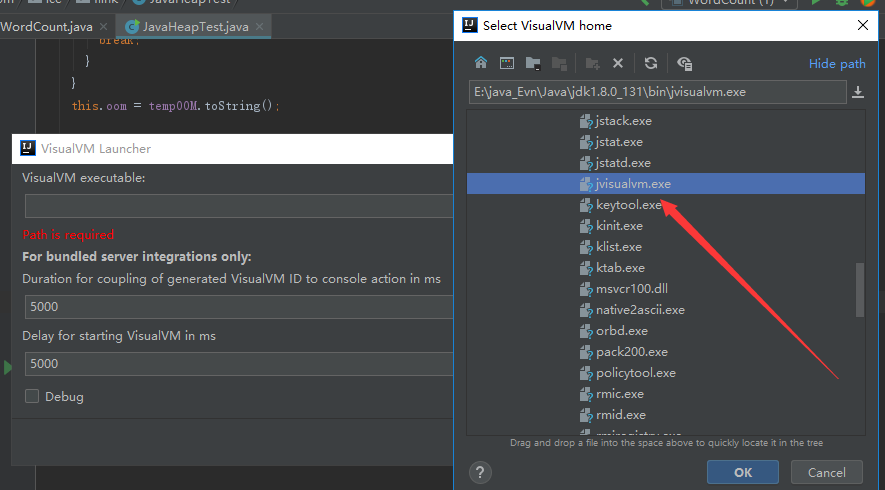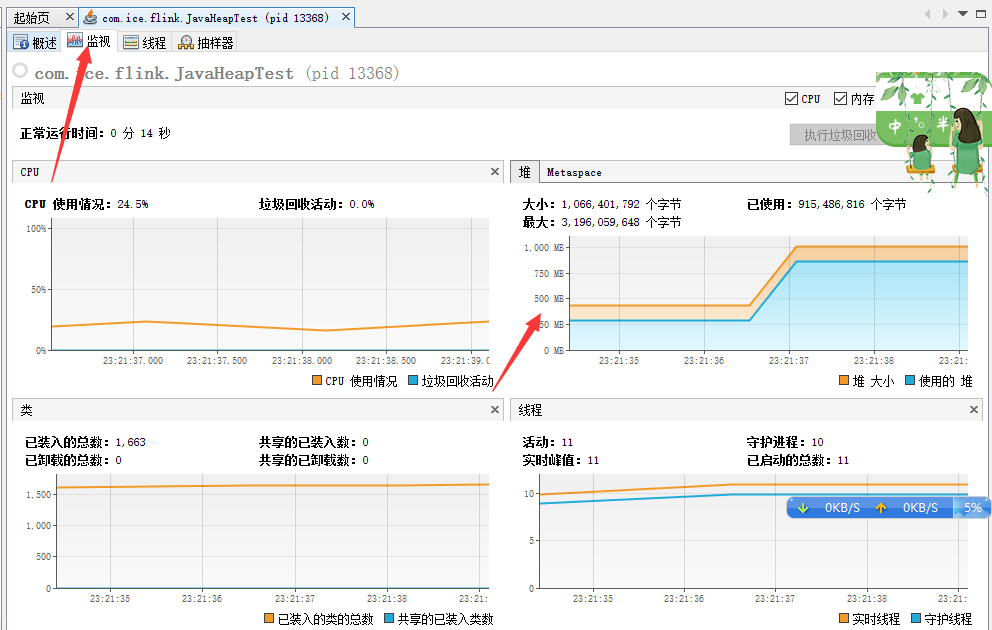上面的图是没有设置任何程序的事情下做的，为什么需要看堆的大小，因为堆存放对象的实例，一般都会需要设置该值，在没有做任何设置的时候，就会看到堆的内存可能达到1G的大小。有时候需要模拟线上环境的上的Java内存大小，在IDEA中设置下一年堆的大小，这里设置堆的初始化和最大值都一样，以避免每次垃圾回收完成后JVM重新分配内存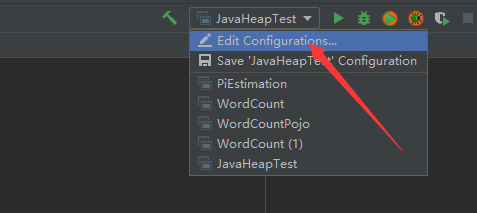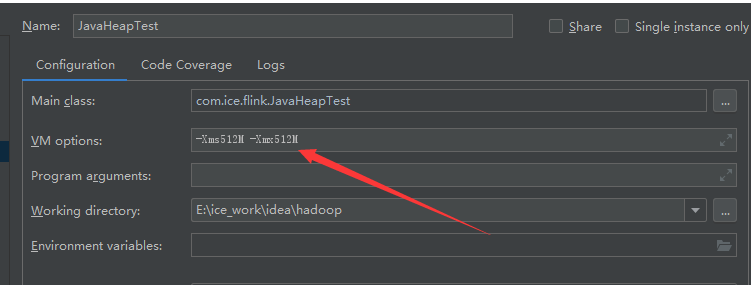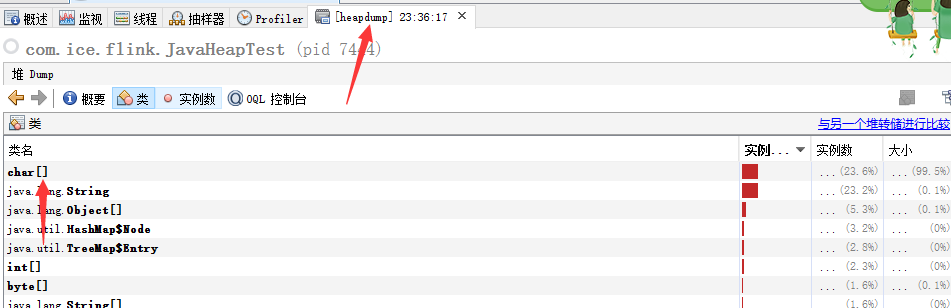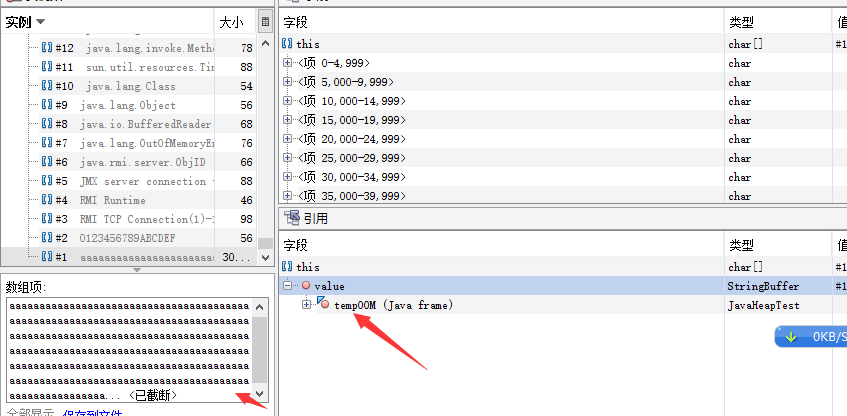## 二、查看程序的线程情况

public class DeadLock {
public static void main(String[] args) {
Resource r1 = new Resource();
Resource r0 = new Resource();

myTh1.start();
myTh0.start();
}
}

class Resource {
private int i;

public int getI() {
return i;
}

public void setI(int i) {
this.i = i;
}

}

private Resource r1, r2;

public LockThread1(Resource r1, Resource r2) {
this.r1 = r1;
this.r2 = r2;
}

@Override
public void run() {
int j = 0;
while (true) {
synchronized (r1) {
System.out.println("The first thread got r1's lock " + j);
synchronized (r2) {
System.out.println("The first thread got r2's lock  " + j);
}
}
j++;
}
}

}

private Resource r1, r2;

public LockThread0(Resource r1, Resource r2) {
this.r1 = r1;
this.r2 = r2;
}

@Override
public void run() {
int j = 0;
while (true) {
synchronized (r2) {
System.out.println("The second thread got r2's lock  " + j);
synchronized (r1) {
System.out.println("The second thread got r1's lock" + j);
}
}
j++;
}
}

}


程序运行之后，查看线程标签后直接告警出现了死锁的线程。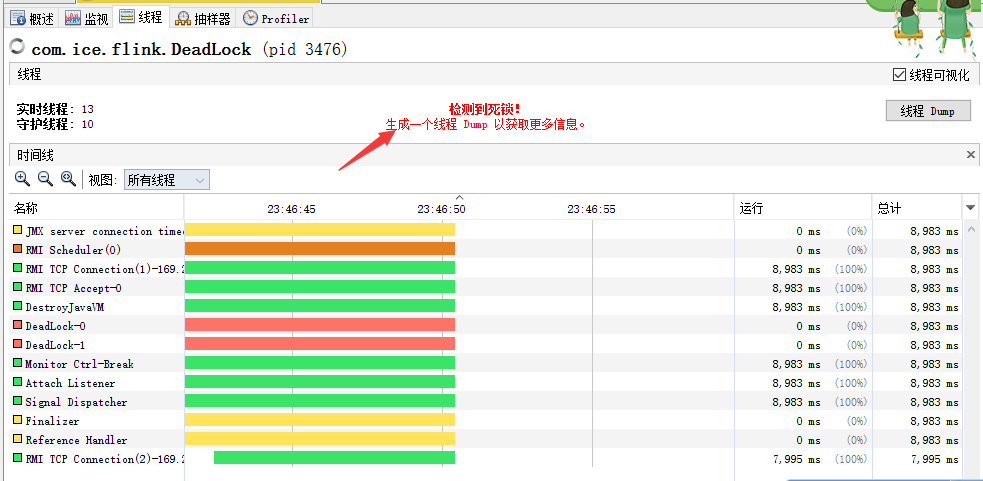JVM调优，需要变设置参数，边进行观察，这样更容易理解其中缘由，可以上手试一试。

posted @ 2018-12-24 23:57  冰魄秋雨  阅读(...)  评论(...编辑  收藏